# Determine, by drawing graphs, whether the following system of linear equations has a unique solution or not :

Question:

Determine, by drawing graphs, whether the following system of linear equations has a unique solution or not :

(i) $2 x-3 y=6, x+y=1$

(ii) $2 y=4 x-6,2 x=y+3$

Solution:

(i) The given equations are

$2 x-3 y=6$$.(i)$

$x+y=1$ $. .(i i)$

Putting $x=0$ in equation $(i)$, we get:

$\Rightarrow 2 \times 0-3 y=6$

$\Rightarrow y=-2$

$x=0, \quad y=-2$

Putting $y=0$ in equation $(i)$ we get:

$\Rightarrow 2 x-3 \times 0=6$

$\Rightarrow x=3$

$x=3, \quad y=0$

Use the following table to draw the graph.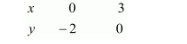Draw the graph by plotting the two points $A(0,-2), B(3,0)$ from table.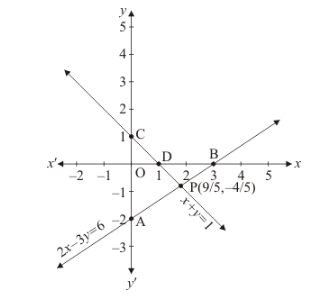Graph of the equation.... (ii):

$x+y=1 \quad \ldots \ldots(i i)$

Putting $x=0$ in equation $(i i)$ we get:

$\Rightarrow 0+y=1$

$\Rightarrow y=1$

$\therefore x=0, \quad y=1$

Putting $y=0$ in equation $(i i)$, we get:

$\Rightarrow x+0=1$

$\Rightarrow x=1$

$x=1, \quad y=0$

Use the following table to draw the graph.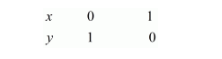Draw the graph by plotting the two points $C(0,1), D(1,0)$ from table.

The two lines intersect at point $P\left(\frac{9}{5},-4 / 5\right)$.

Hence the equations have unique solution.

(ii) The equations of graphs is

$2 y=4 x-6$

$4 x-2 y=6$...$(i)$

$2 x=y+3$

$2 x-y=3$..$.(i i)$

Putting $x=0$ in equation $(i)$, we get:

$\Rightarrow 4 x-2 \times 0=6$

$\Rightarrow x=3 / 2$

$x=3 / 2, \quad y=0$

Use the following table to draw the graph.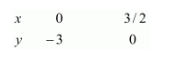The graph of $(i)$ can be obtained by plotting the two points $A(0,-3), B(3 / 2,0)$.

Graph of the equation (ii)

$2 x-y=3$.... (ii)

Putting $x=0$ in equation $(i i)$, we get.

$\Rightarrow 2 x-0=3$

$\Rightarrow x=3 / 2$

$x=3 / 2, \quad y=0$

Use the following table to draw the graph.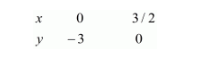Draw the graph by plotting the two points $C(0,-3), D(3 / 2,0)$ from table.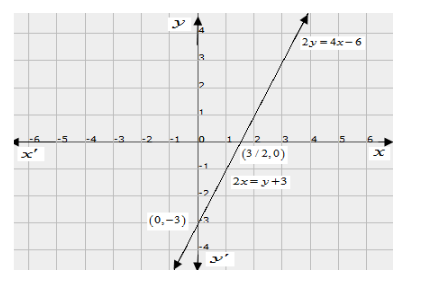The two lines are coincident.

Hence the equations have infinitely much solution.

Hence the system is consistent